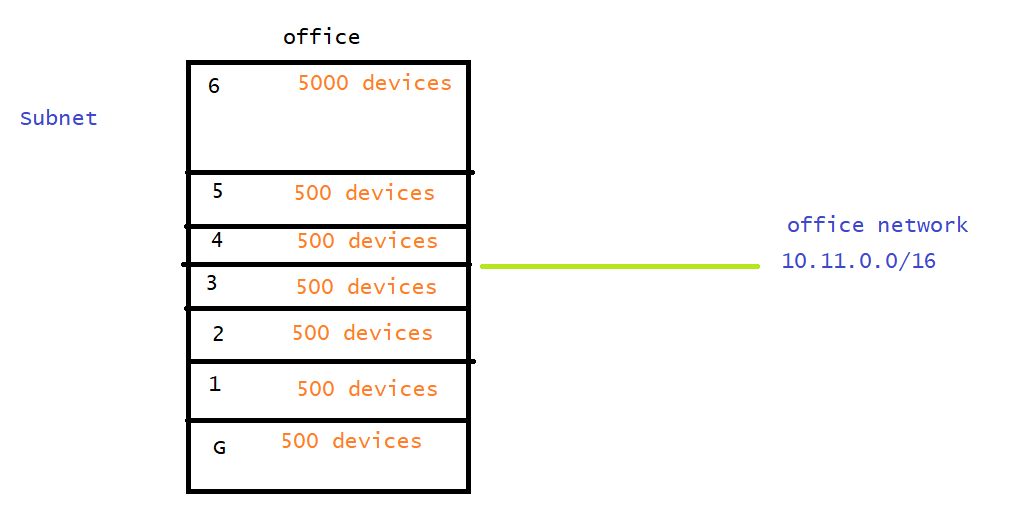### Networking contd..

• Create a network which can help in connecting 2000 devices
• the host id size should connect to 2000 devices
• 2^n ~= 2000 answer would n is 11 as 2^11 = 2048
• Now host size would be 11 bits
• What should be ip addresss range? It is better to use private network cidr ranges
• Private cidr ranges
• 10.0.0.0/8
• 172.16.0.0/12
• 192.168.0.0/16
• Lets choose any one of the above ranges
``````iprange: 192.168.0.0/21
255.255.248.0
``````
• What would be ip address range for connecting to 3000 devices on your network?
``````2^n ~= 3000 n = 12
ip range: 172.16.0.0/20
255.255.240.0
``````
• What would be ip address range for connecting to 100000 devices on your network
``````2^n ~= 100000  n = 17
ip range: 10.0.0.0/15
255.254.0.0
``````
• Generally we would not work with one complete network, we try to break network into smaller sub networks and these smaller networks are called as subnets.

#### Networks and Subnets

• Lets try to create subnets from a network
``````network ip range: 192.168.0.0/16
network id: 192.168
size = 65000

lets try to create a subnet which can connect to 200 devices
2^n ~= 200 n = 8

ip range: 192.168.0.0/24
network id 192.168.0
size = 250

lets create two more subnets which can connect 200 devices each
192.168.00000001.00000000
subnet 2: 192.168.1.0/24
192.168.00000010.00000000
subnet 3: 192.168.2.0/24
``````
• Lets try to create subnets from a network
``````network ip range: 10.10.0.0/16

now lets create two subnets which can connect to 1000 devices
2^n ~= 1000 n = 10

= 255.255.252.0

``````
• Lets create subnets (subnet1, subnet2) which can connect to 200 and 500 devices
``````ip address range: 172.16.0.0/16

subnet1 : 172.16.0.0/24
2^n ~= 200 n = 8

subnet2:
2^n ~= 500 n = 9
172.16.0000001   0.00000000 => 172.16.2.0/23
255.255.254.0

``````
• Try to calculate subnet cidr ranges for the office network shown belowThis site uses Akismet to reduce spam. Learn how your comment data is processed.